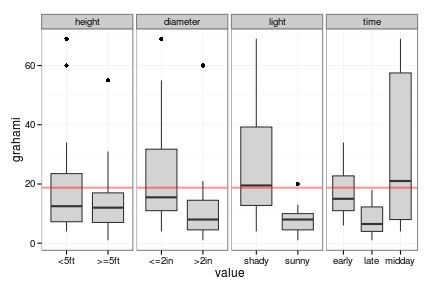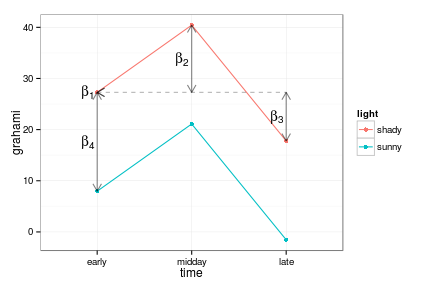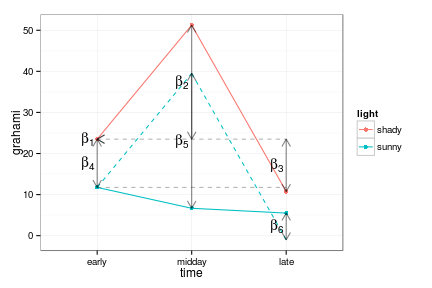## Interpreting parameters of linear models

### Coding for categorical predictors: contrasts

• What do the parameters of a linear model mean?
• Start with categorical variables, because they're potentially more confusing (“intercept and slope” isn't too hard)
• Default R behaviour: treatment contrasts. $$\beta_1$$ = expected value in baseline group (= first level of the factor variable, by default the first in alphabetical order); $$\beta_i$$ = expected difference between group $$i$$ and the first group.

For example, if we took the ants example from our permutations and ran a linear model, the results would look like this:

pr <- function(m) printCoefmat(coef(summary(m)),digits=3,signif.stars=FALSE)
pr(lm1 <- lm(colonies~place,data=ants))

##             Estimate Std. Error t value Pr(>|t|)
## (Intercept)    10.75       0.98   10.97  4.2e-06
## placeforest    -3.75       1.27   -2.96    0.018


(pr is just a utility function I made up for printing compact summaries of the estimates from a linear regression: summary() would give the same information plus a lot more I'm not interested in right now.) The (Intercept) row refers to $$\beta_1$$, which is the mean density in the “field” sites (“field” comes before “forest”). The placeforest row tells us we are looking at the effect of the place variable on the forest level, i.e. the difference between the “forest” and “field” sites. (The only ways we could know that “field” is the baseline site are (1) to remember, or look at levels(ants$place) or (2) to notice which level is missing from the list of parameter estimates.) R's behaviour may seem annoyng at first – it seems like the estimated values of the groups are what we're really interested in – but it is really designed for testing differences among groups. To get the estimates per group (what SAS calls LSMEANS), you could: • use a regression formula colonies~place-1, or equivalently colonies~place+0, to suppress the implicit intercept term: ## Estimate Std. Error t value Pr(>|t|) ## placefield 10.75 0.98 10.97 4.2e-06 ## placeforest 7.00 0.80 8.75 2.3e-05  • Use the predict function: predict(lm1,newdata=data.frame(place=c("field","forest")),interval="confidence")  • Use the effects package: library("effects") summary(allEffects(lm1))  You can (and should) look at graphical summaries of your results via plot(allEffects(lm1))  or library("coefplot2") coefplot2(lm0,intercept=TRUE)  (or coefplot2(lm1)). When you use the colonies~place-1 formula, the meanings of the parameters change: $$\beta_1$$ is the same (mean of “field”), but $$\beta_2$$ is 'mean of “field”' rather than (“(field)-(forest)”). Things get slightly more interesting/complicated when we have more than two levels of a categorical variable. I'll look at some data on lizard perching behaviour, from the brglm package (and before that from McCullagh and Nelder Generalized Linear Models 1989, ultimately from Schoener 1970 Ecology 51:408-418). I'm going to ignore the fact that these data might best be fitted with generalized linear models. lizards <- read.csv("lizards.csv")  A quick look at the data: response is number of Anolis grahami lizards found on a perches in particular conditions.For a moment we're going to just look at the time variable. If we leave the factors as is (alphabetical) then $$\beta_1$$=“early”, $$\beta_2$$=“late”-“early”, $$\beta_3$$=“midday”-“early”. At the very least, it probably makes sense to change the order of the levels: lizards$time <- factor(lizards\$time,levels=c("early","midday","late"))


All this does (since we haven't changed the baseline factor) is swap the definitions of $$\beta_2$$ and $$\beta_3$$.

In a linear model, we could also use so-called sum-to-zero contrasts:

pr(lm(grahami~time,data=lizards,contrasts=list(time=contr.sum)))

##             Estimate Std. Error t value Pr(>|t|)
## (Intercept)    19.30       3.53    5.47  2.4e-05
## time1          -1.67       4.93   -0.34     0.74
## time2          12.85       5.10    2.52     0.02


Now the (Intercept) parameter is the overall mean: time1 and time2 are the deviations of the first (“early”) and second (“midday”) groups from the overall mean. (The names are useless: the car package offers a slightly better alternative called contr.Sum). There are other ways to change the contrasts (i.e., use the contrasts() function to change the contrasts for a particular variable permanently, or use options(contrasts=c("contr.sum","contr.poly"))) to change the contrasts for all variables), but the way shown above may be the most transparent.

There are other options for contrasts such as MASS::contr.sdif(), which gives the successive differences between levels.

library("MASS")
pr(lm(grahami~time,data=lizards,contrasts=list(time=contr.sdif)))

##             Estimate Std. Error t value Pr(>|t|)
## (Intercept)    19.30       3.53    5.47  2.4e-05
## time2-1        14.52       8.74    1.66    0.112
## time3-2       -24.02       8.74   -2.75    0.012


You might have particular contrasts in mind (e.g. “control” vs. all other treatments, then “low” vs “high” within treatments), in which case it is probably worth learning how to set contrasts. (We will talk about testing all pairwise differences later, when we discuss multiple comparisons. This approach is probably not as useful as it is common.)

## Multiple treatments and interactions

Let's consider the light variable in addition to time.

pr(lmTL1 <- lm(grahami~time+light,data=lizards))

##             Estimate Std. Error t value Pr(>|t|)
## (Intercept)    27.29       5.63    4.85  0.00011
## timemidday     13.14       7.11    1.85  0.08010
## timelate       -9.50       6.85   -1.39  0.18174
## lightsunny    -19.32       5.73   -3.37  0.00321


Here's a graphical interpretation of the parameters:$$\beta_1$$ is the intercept (“early”,“sunny”); $$\beta_2$$ and $$\beta_3$$ are the differences from the baseline level (“early”) of the first variable (time) in the baseline level of the other parameter(s) (light=“shady”); $$\beta_4$$ is the difference from the baseline level (“sunny”) of the second variable (light) in the baseline level of time (“early”).

Now let's look at an interaction model:

pr(lmTL2 <- lm(grahami~time*light,data=lizards))

##                       Estimate Std. Error t value Pr(>|t|)
## (Intercept)              23.50       5.38    4.37  0.00042
## timemidday               27.75       7.60    3.65  0.00198
## timelate                -12.75       7.60   -1.68  0.11180
## lightsunny              -11.75       7.60   -1.55  0.14061
## timemidday:lightsunny   -32.83      11.19   -2.93  0.00927
## timelate:lightsunny       6.50      10.75    0.60  0.55343Parameters $$\beta_1$$ to $$\beta_4$$ have the same meanings as before. Now we also have $$\beta_5$$ and $$\beta_6$$, labelled “timemidday:lightsunny” and “timelate:lightsunny”, which describe the difference between the expected mean value of these treatment combinations based on the additive model (which are $$\beta_1 + \beta_2 + \beta_4$$ and $$\beta_1 + \beta_3 + \beta_4$$ respectively) and their actual values.

Now re-do this for sum-to-zero contrasts … the fits are easy:

pr(lmTL1S <- update(lmTL1,contrasts=list(time=contr.sum,light=contr.sum)))

##             Estimate Std. Error t value Pr(>|t|)
## (Intercept)    18.84       2.87    6.57  2.7e-06
## time1          -1.21       4.01   -0.30   0.7654
## time2          11.93       4.15    2.87   0.0097
## light1          9.66       2.87    3.37   0.0032

pr(lmTL2S <- update(lmTL2,contrasts=list(time=contr.sum,light=contr.sum)))

##              Estimate Std. Error t value Pr(>|t|)
## (Intercept)    18.236      2.255    8.09  3.1e-07
## time1          -0.611      3.146   -0.19  0.84830
## time2          10.722      3.271    3.28  0.00444
## light1         10.264      2.255    4.55  0.00028
## time1:light1   -4.389      3.146   -1.39  0.18100
## time2:light1   12.028      3.271    3.68  0.00187


(The intercept doesn't stay exactly the same when we add the interaction because the data are unbalanced: try with(lizards,table(light,time)))

The pictures will be a pain – maybe draw by hand?

Other refs

library("wwiki")
options(MWUser="Bb")
pushWiki("contrasts2.rmd",page="linear model parameters",wiki="bio_708")